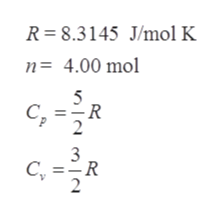You are conducting experiments to study prototype heat engines. In one test, 4.00 mol of argon gas are taken around the cycle shown in. The pressure is low enough for the gas to be treated as ideal. You measure the gas temperature in states a, b, c, and d and find Ta = 250.0˚K, Tb = 300.0˚K, Tc = 380.0˚K, andTd = 316.7˚K.A to B -- constant volumeB to C -- constant pressureC to D -- constant volume D to A -- constant pressure  Calculate the efficiency e of the cycle. e = ______ %

Question

You are conducting experiments to study prototype heat engines. In one test, 4.00 mol of argon gas are taken around the cycle shown in. The pressure is low enough for the gas to be treated as ideal. You measure the gas temperature in states a, b, c, and d and find Ta = 250.0˚K, Tb = 300.0˚K, Tc = 380.0˚K, andTd = 316.7˚K.

A to B -- constant volume

B to C -- constant pressure

C to D -- constant volume

D to A -- constant pressure

Calculate the efficiency e of the cycle.

e = ______ %

Step 1

The P-V diagram for the question will be as follows:

Step 2

Given,

Now, for a given monoatomic ideal gas, we have specific heat at constant pressure (Cp) and at constant volume (Cv) and where R is the gas constant and n is the number of moles as:help_outlineImage TranscriptioncloseR 8.3145 J/mol K n= 4.00 mol 5 -R 2 3 C, R 2 fullscreen
Step 3

Plugging the above given values in Q for an isochoric process A→...

Want to see the full answer?

See Solution

Want to see this answer and more?

Our solutions are written by experts, many with advanced degrees, and available 24/7

See Solution
Tagged in

Thermodynamics